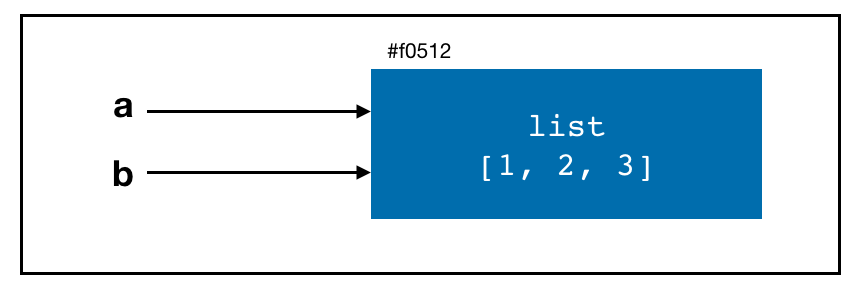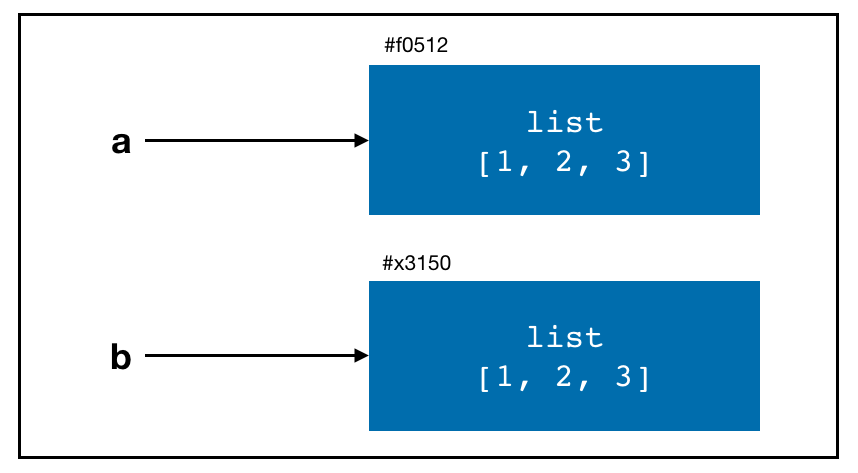In this lecture, we will discuss:

• Python semantics
• Object-oriented programming
• Variables and argument passing

## 1. Indentations, not braces

• Other languagues (R, C++, Java, etc.) use braces to structure code.
a = 10
if (a < 5) {
result = TRUE
b = 0
} else {
result = FALSE
b = 100
}
• Python uses whitespaces (tabs or spaces) to structure code instead.
a = 10
if a < 5:
result = True
b = 0
else:
result = False
b = 100
• A colon : denotes the start of an indented code block.
• Python statements (like R statements) do not need to be separated by semicolons ;.
• Recommended: Use TAB for indentation.
• One of the most common errors in Python is incorrect indentation.
• Be consistent with how you indent your code goes a long way!

## 2. Everything is an object

• Python is an object-oriented programming language (so is R). That is, every number, string, data structure, function, etc. is a Python object.
• Think of them as a BOX. There are “doors” (methods) associated with each box to help us access the content of the box.
• Each object has an associated type (like string, list, DataFrame, etc.).

• Use hashtag # to denote a comment in Python (just like R!).
# initialize count
count = 0

for i in [1, 2, 3]:
# increase count
count = count + 1

## 4. Variables and arguments passing

• When assigning an object to a variable in Python, you are creating a reference to the object on the righthand side of the equals sign.
a = [1, 2, 3]
b = a
• In Python, a and b actually now refer to the same object, the original list [1, 2, 3].• In some languages, this assignment would cause the data [1, 2, 3] to be copied.## 5. Dynamic references, strong types

• Object references (variables) in Python have no type associated with them.
a = 5
type(a)
## <class 'int'>
a = 'foo'
type(a)
## <class 'str'>

## 6. Attributes and methods

• Objects in Python typically have both:
• Attributes - other Python objects stored “inside” the object.
• Methods - functions associated with an object that can have access to the object’s internal data.
• They can be accessed via the syntax obj.attribute_name or obj.method_name.

## 7. Imports

• In Python a module (or library) is simply a file with the .py extension containing Python code.
# some_module.py
PI = 3.14159

def f(x):
return x + 2

def g(a, b):
return a + b

If we want to access the variables and functions defined in some_module.py:

import some_module

result = some_module.f(5)
pi = some_module.PI

Or

from some_module import f, g, PI

result = g(f(5), PI)

Or

import some_module as sm
from some_module import PI as pi, g as gf

r1 = sm.f(pi)
r2 = gf(r1, pi)

## 8. Binary operators and comparisons

Operation Description
a + b Add a and b
a - b Substract b from a
a * b Multiply a by b
a / b Divide a by b
a // b Floor-divide a by b, dropping any fractional remainder
a ** b Raise a to the b power
a & b True if both a and b are True; for integers, take the bitwise AND
a | b True if either a or b is True; for integers, take the bitwise OR
a ^ b True if a is True or b is True, BUT NOT BOTH; for integers, take the bitwise EXCLUSIVE-OR
a == b True if a equals b
a != b True if a is not equal to b
a <= b, a < b True if a is less than (less than or equal) to b
a >= b, a > b True if a is greater than (greater than or equal) to b
a is b True if a and b reference the same Python object
a is not b True if a and be references different Python objects
• Comparing with is is NOT the same as the == operator:
a = [1, 2, 3]
b = a
c = list(a)
a is b
## True
a is c
## False
a == c
## True

## 9. Mutable and immutable objects

• Most objects in Python (list, dict, DataFrame) are mutable, meaning the object or values that they contain can be modified.
a_list = ['foo', 2, [4, 5]]
a_list = (3, 4)
a_list
## ['foo', 2, (3, 4)]
• Other objects, like strings or tuples, are immutable.
a_tuple = (3, 5, (2, 4))
a_tuple = 2
## Error in py_call_impl(callable, dots$args, dots$keywords): TypeError: 'tuple' object does not support item assignment
##
## Detailed traceback:
##   File "<string>", line 1, in <module>

This lecture note is modified from Chapter 2 of Wes McKinney’s Python for Data Analysis 2nd Ed.